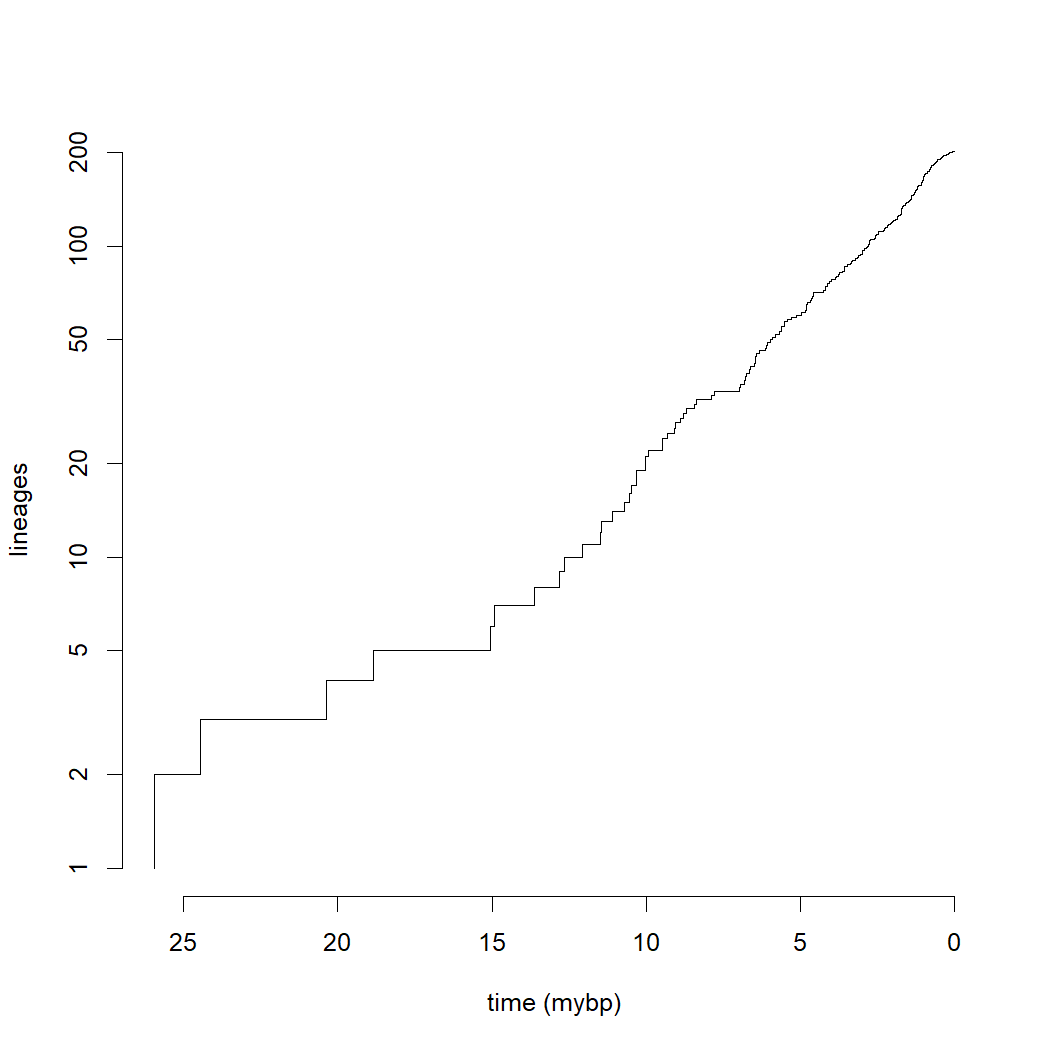## Tuesday, May 17, 2022

### A quick & easy trick to flip the x ("time") axis on a lineages through time plot

Here's a quick & easy trick to flip the direction of the x-axis (to show time running backwards from the present day into the past) on a lineages-through-time (LTT) plot.

To start, I'll load phytools and read a tree from file. (Here I'm using a tree of darters from Near et al. 2011.)

``````library(phytools)
"http://www.phytools.org/Rbook/9/etheostoma_percina_chrono.tre")
``````

Next, I'll compute my `"ltt"` object without graphing it using `phytools::ltt`.

``````obj<-ltt(tree,plot=FALSE)
obj
``````
``````## Object of class "ltt" containing:
##
## (1) A phylogenetic tree with 201 tips and 198 internal
##     nodes.
##
## (2) Vectors containing the number of lineages (ltt) and
##     branching times (times) on the tree.
##
## (3) A value for Pybus & Harvey's "gamma" statistic of
##     gamma = NA, p-value = NA.
``````

(The γ statistic shows up as `NA` because our tree has polytomies – but for graphing the LTT we don't need to worry about that.)

Finally, I'll graph the `"ltt"` object, without showing the axes, I'll add, first, the vertical (y) axis – and then I'll flip the directionality of the x axis before adding it too.

``````plot(obj,log="y",log.lineages=FALSE,bty="n",axes=FALSE,
xlab="time (mybp)")
axis(2)
h<-axTicks(1)
par(usr=c(par()\$usr[2:1],par()\$usr[3:4]))
axis(1,h[length(h):1])
``````Cool. It works!